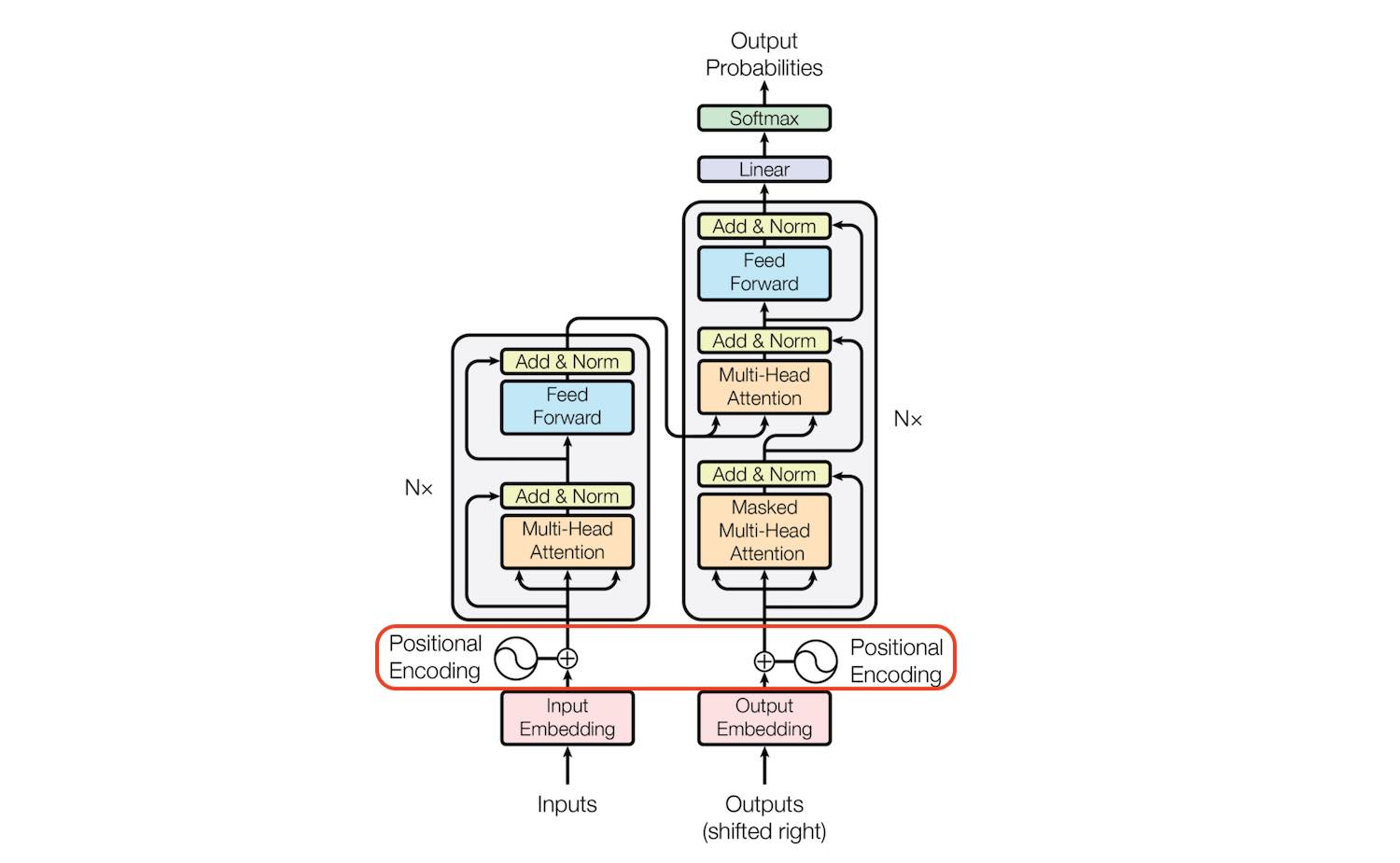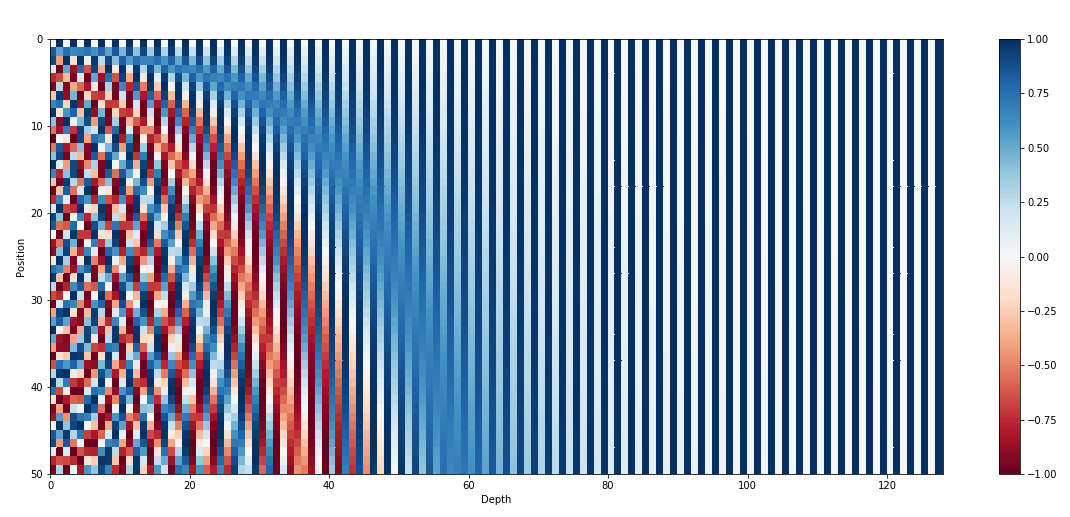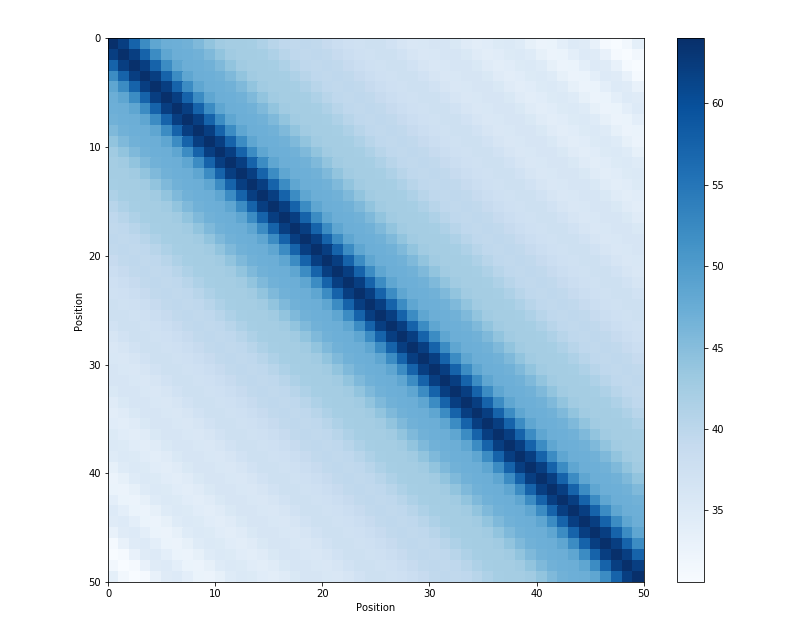# Transformer Architecture: The Positional Encoding

## Let's use sinusoidal functions to inject the order of words in our model

Posted by September 20, 2019 · 17 mins read

Transformer architecture was introduced as a novel pure attention-only sequence-to-sequence architecture by Vaswani et al. Its ability for parallelizable training and its general performance improvement made it a popular option among NLP (and recently CV) researchers.

Thanks to the several implementations in common deep learning frameworks, it became an easy option to experiment with for many students (including myself). Even though making it more accessible is a great thing, but on the downside it may cause the details of the model to be ignored.

In this article, I don’t plan to explain its architecture in depth as there are currently several great tutorials on this topic (here, here, and here), but alternatively, I want to discuss one specific part of the transformer’s architecture - the positional encoding.

When I read this part of the paper, it raised some questions in my head, which unfortunately the author had not provided sufficient information to answer them. So in this article, I want to try to break this module apart and look at how it works.

NOTE: To understand the rest of this post, I highly suggest you read one those tutorials to get familiar with the transformer architecture.Figure 1 - The Transformer Architecture

Header Photo by Susan Yin on Unsplash

## What is positional encoding and Why do we need it in the first place?

Position and order of words are the essential parts of any language. They define the grammar and thus the actual semantics of a sentence. Recurrent Neural Networks (RNNs) inherently take the order of word into account; They parse a sentence word by word in a sequential manner. This will integrate the words’ order in the backbone of RNNs.

But the Transformer architecture ditched the recurrence mechanism in favor of multi-head self-attention mechanism. Avoiding the RNNs’ method of recurrence will result in massive speed-up in the training time. And theoretically, it can capture longer dependencies in a sentence.

As each word in a sentence simultaneously flows through the Transformer’s encoder/decoder stack, The model itself doesn’t have any sense of position/order for each word. Consequently, there’s still the need for a way to incorporate the order of the words into our model.

One possible solution to give the model some sense of order is to add a piece of information to each word about its position in the sentence. We call this “piece of information”, the positional encoding.

The first idea that might come to mind is to assign a number to each time-step within the [0, 1] range in which 0 means the first word and 1 is the last time-step. Could you figure out what kind of issues it would cause? One of the problems it will introduce is that you can’t figure out how many words are present within a specific range. In other words, time-step delta doesn’t have consistent meaning across different sentences.

Another idea is to assign a number to each time-step linearly. That is, the first word is given “1”, the second word is given “2”, and so on. The problem with this approach is that not only the values could get quite large, but also our model can face sentences longer than the ones in training. In addition, our model may not see any sample with one specific length which would hurt generalization of our model.

Ideally, the following criteria should be satisfied:

• It should output a unique encoding for each time-step (word’s position in a sentence)
• Distance between any two time-steps should be consistent across sentences with different lengths.
• Our model should generalize to longer sentences without any efforts. Its values should be bounded.
• It must be deterministic.

## Proposed method

The encoding proposed by the authors is a simple yet genius technique which satisfies all of those criteria. First of all, it isn’t a single number. Instead, it’s a $d$-dimensional vector that contains information about a specific position in a sentence. And secondly, this encoding is not integrated into the model itself. Instead, this vector is used to equip each word with information about its position in a sentence. In other words, we enhance the model’s input to inject the order of words.

Let $t$ be the desired position in an input sentence, $\vec{p_t} \in \mathbb{R}^d$ be its corresponding encoding, and $d$ be the encoding dimension (where $d \equiv_2 0$) Then $f : \mathbb{N} \rightarrow \mathbb{R}^d$ will be the function that produces the output vector $\vec{p_t}$ and it is defined as follows:

where

As it can be derived from the function definition, the frequencies are decreasing along the vector dimension. Thus it forms a geometric progression from $2\pi$ to $10000 · 2\pi$ on the wavelengths.

You can also imagine the positional embedding $\vec{p_t}$ as a vector containing pairs of sines and cosines for each frequency (Note that $d$ is divisble by 2):

## The intuition

You may wonder how this combination of sines and cosines could ever represent a position/order? It is actually quite simple, Suppose you want to represent a number in binary format, how will that be?

You can spot the rate of change between different bits. The LSB bit is alternating on every number, the second-lowest bit is rotating on every two numbers, and so on.

But using binary values would be a waste of space in the world of floats. So instead, we can use their float continous counterparts - Sinusoidal functions. Indeed, they are the equivalent to alternating bits. Moreover, By decreasing their frequencies, we can go from red bits to orange ones.Figure 2 - The 128-dimensional positonal encoding for a sentence with the maximum lenght of 50. Each row represents the embedding vector $\vec{p_t}$

## Other details

Earlier in this post, I mentioned that positional embeddings are used to equip the input words with their positional information. But how is it done? In fact, the original paper added the positional encoding on top of the actual embeddings. That is for every word $w_t$ in a sentence $[w_1,...w_n]$, Calculating the correspondent embedding which is fed to the model is as follows:

To make this summation possible, we keep the positional embedding’s dimension equal to the word embeddings’ dimension i.e. $d_\text{word embedding} = d_\text{postional embedding}$

## Relative Positioning

Another characteristic of sinusoidal positional encoding is that it allows the model to attend relative positions effortlessly. Here is a quote from the original paper:

We chose this function because we hypothesized it would allow the model to easily learn to attend by relative positions, since for any fixed offset k, PEpos+k can be represented as a linear function of PEpos.

But why does this statement hold? To fully understand why, please refer to this great article to read the detailed proof. However I’ve prepared a shorter version here.

For every sine-cosine pair corresponding to frequency $\omega_k$, there is a linear transformation $M \in \mathbb{R}^{2\times2}$ (independent of $t$) where the following equation holds:

Proof:

Let $M$ be a $2 \times 2$ matrix, we want to find $u_1, v_1, u_2$ and $v_2$ so that:

By applying the addition theorem, we can expand the right hand side as follows:

Which result in the following two equations:

By solving above equations, we get:

So the final transformation matrix $M$ is:

As you can see, the final transformation does not depend on $t$. Note that one can find the matrix $M$ very similar to the rotation matrix.

Similarly, we can find $M$ for other sine-cosine pairs, which eventually allows us to represent $\vec{p_{t+\phi}}$ as a linear function of $\vec{p_t}$ for any fixed offset $\phi$. This property, makes it easy for the model to learn to attend by relative positions.

Another property of sinusoidal position encoding is that the distance between neighboring time-steps are symmetrical and decays nicely with time.Figure 3 - Dot product of position embeddings for all time-steps

## FAQ

### Why positional embeddings are summed with word embeddings instead of concatenation?

I couldn’t find any theoretical reason for this question. Since summation (in contrast to concatenation) saves the model’s parameters, it is reasonable to reform the initial question to “Does adding the positional embeddings to words have any disadvantages?”. I would say, not necessarily!

Initially, if we pay attention to the figure 2, we will find out that only the first few dimensions of the whole embedding are used to store the information about the positions (Note that the reported embedding dimension is 512 despite our small toy example). And since the embeddings in the Transfomer are trained from scratch, the parameters are probably set in a way that the semantic of words does not get stored in the first few dimensions to avoid interfering with the positional encoding.

With the same reason, I think the final Transformer can separate the semantic of words from their positional information. Moreover, there is no reason to consider the separability as an advantage. Maybe the summation provides a good source of feature for the model to learn from.

### Doesn't the position information get vanished once it reaches the upper layers?

Fortunately, the Transformer architecture is equipped with residual connections. Therefore the information from the input of the model (which contains positional embeddings) can efficiently propagate to further layers where the more complex interactions are handled.

### Why are both sine and cosine used?

Personally, I think, only by using both sine and cosine, we can express the sine(x+k) and cosine(x+k) as a linear transformation of sin(x) and cos(x). It seems that you can’t do the same thing with the single sine or cosine. If you can find a linear transformation for a single sine/cosine, please let me know in the comments section.

## Summary

Thank you for staying with me until the end of this article. I hope you’ve found this useful for answering your question. Please feel free to provide any corrections or feedbacks, the comment section is at your disposal.

Cited as

@article{kazemnejad2019:pencoding,
title   = "Transformer Architecture: The Positional Encoding",